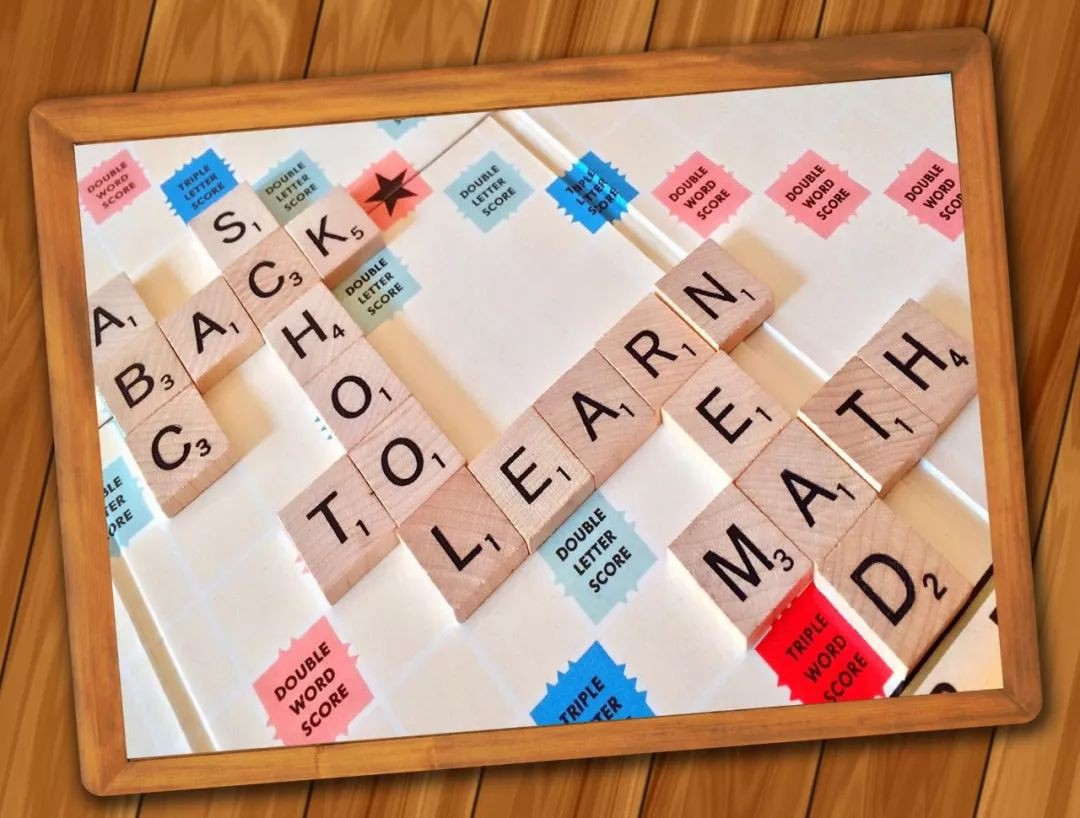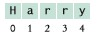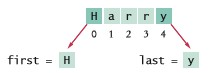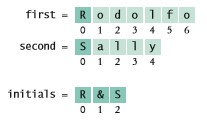# 手把手教你學Python：一文看懂字串基本操作01 字串型別

``````print("Hello")
``````

``````greeting = "Hello"
``````

``````print(greeting)
``````

``````print("This is a string.", 'So is this.')
``````

``````message = 'He said "Hello" '
``````

``````length = len("World!")   # 長度為6
``````

``````""
``````

``````''
``````

02 連接與重覆

``````firstName = "Harry"
lastName = "Morgan"
name = firstName + lastName
``````

``````"HarryMorgan"
``````

``````name = firstName + " " + lastName
``````

``````"Harry Morgan"
``````

``````dashes = "-" * 50
``````

``````"-------------------------------------------------"
``````

``````message = "Echo..."
print(message * 5)
``````

``````Echo...Echo...Echo...Echo...Echo...
``````

03 轉換數字和字串

``````name = "Agent " + 1729   # 錯誤：只能連接字串
``````

``````str(1729)
``````

``````id = 1729
name = "Agent " + str(id)
``````

``````id = int("1729")
price = float("17.29")
``````

``````value = float("17x29")
``````

``````int("　1729　")
``````

04 字串與字符``````name = "Harry"
``````

``````first = name
last = name
````````````pos = len(name) - 1   # "Harry"的長度是5
last = name[pos]      # last被設置為"y"
``````▲圖2-4 創建首字母字串

``````##
#  本程式用來輸出一對首字母
#

# 設置兩個名字
first = "Rodolfo"
second = "Sally"

# 計算和顯示首字母
initials = first + "&" + second
print(initials)
``````

 陳述句 結果 說明 string = “Py” string = string + “thon” string被設置位”Python” 當作用於字串時，+表示連接。 print(“Please” + ” enter your name: “) 打印 Please enter your name: 使用續行符打斷無法在一行內編寫的字串。 team = str(49) + “ers” team被設置為”49ers” 因為49是一個整數，必須被轉換位字串。 greeting = “H & S” n = len(greeting) n被設置為5 在統計數量時每個空格都作為一個字符。 string = “Sally” ch = string ch被設置為”a” 註意，第一個下標是0。 last =   string[len(string) – 1] last被設置為包含string中最後一個字符的字串 最後一個字符的位置是len(string)   – 1。

05 字串方法

``````name = "John Smith"
uppercaseName = name.upper()   # 把uppercaseName設置為"JOHN SMITH"
``````

``````print(name.lower())   # 輸出john smith
``````

``````name2 = name.replace("John", "Jane")   # 把name2設置為"Jane Smith"
``````

• s.lower()：字串s的小寫版本

• s.upper()：字串s的大寫版本

• s.replace(old, new)：傳回一個新字串，其中子字串old在s中的每次出現都被替換為字串new